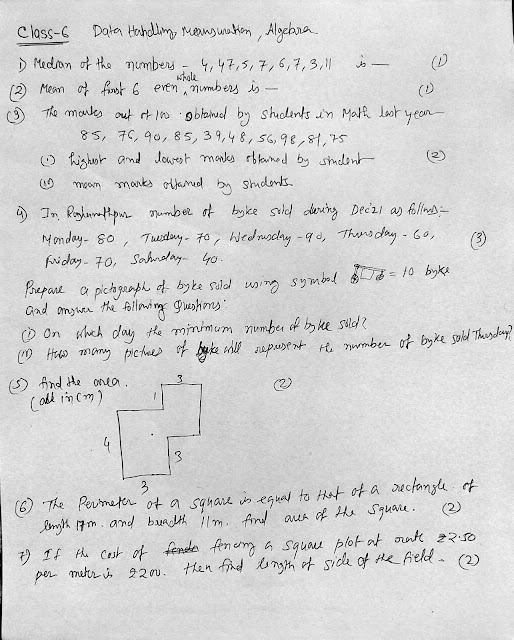30 May 2019

ABCD is a Parallelogram in which AP bisector angle A and BQ bisect angle B. Prove that AQ=BP , PQ= CD. ABPQ is a parallelogram, SELINA class 9 math ex 14 C question 4

ABCD is a Parallelogram in which AP bisector angle A and BQ bisect angle B.
Prove that (i)AQ=BP
(ii) PQ= CD
(iii) ABPQ is a parallelogram

In parallelogram ABCD, P is mid-point of AB. CP and BD intersect each other at point O. If area of POB = 40 cm^2, and OP : OC = 1:2, find: (i) Areas of BOC and PBC (ii) Areas of ABC and parallelogram ABCD.

1.1.2.Thank you so much sir

3.Thank you so much sir